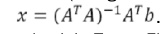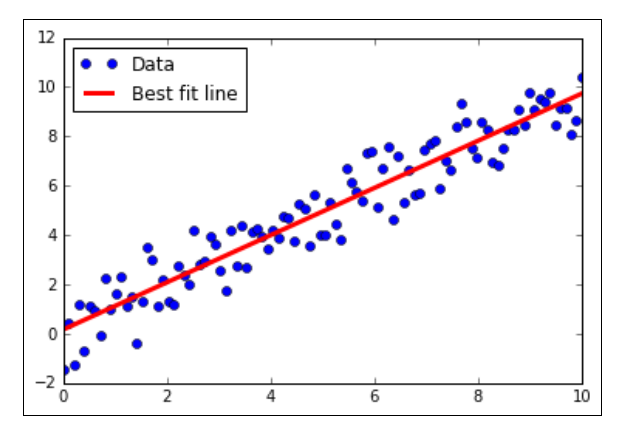1604308368

# Linear regression with TensorFlow

Linear regression may be one of the most important algorithms in statistics, machine learning, and science in general. It’s one of the most used algorithms and it is very important to understand how to implement it and its various flavours. One of the advantages that linear regression has over many other algorithms is that it is very interpretable.

### Using the Matrix Inverse Method

we will use TensorFlow to solve two-dimensional linear regressions with the

matrix inverse method.

Linear regression can be represented as a set of matrix equations, say Ax =B. Here we are interested in solving the coefficients in matrix x. We have to be careful if our observation matrix (design matrix) A is not square. The solution to solving x can be expressed asTo show this is indeed the case, we will generate two-dimensional data,

solve it in TensorFlow, and plot the result.

#data-science #artificial-intelligence #machine-learning #tensorflow

## Buddha Community1594271340

## A Deep Dive into Linear Regression

Let’s begin our journey with the truth — machines never learn. What a typical machine learning algorithm does is find a mathematical equation that, when applied to a given set of training data, produces a prediction that is very close to the actual output.

Why is this not learning? Because if you change the training data or environment even slightly, the algorithm will go haywire! Not how learning works in humans. If you learned to play a video game by looking straight at the screen, you would still be a good player if the screen is slightly tilted by someone, which would not be the case in ML algorithms.

However, most of the algorithms are so complex and intimidating that it gives our mere human intelligence the feel of actual learning, effectively hiding the underlying math within. There goes a dictum that if you can implement the algorithm, you know the algorithm. This saying is lost in the dense jungle of libraries and inbuilt modules which programming languages provide, reducing us to regular programmers calling an API and strengthening further this notion of a black box. Our quest will be to unravel the mysteries of this so-called ‘black box’ which magically produces accurate predictions, detects objects, diagnoses diseases and claims to surpass human intelligence one day.

We will start with one of the not-so-complex and easy to visualize algorithm in the ML paradigm — Linear Regression. The article is divided into the following sections:

1. Need for Linear Regression

2. Visualizing Linear Regression

3. Deriving the formula for weight matrix W

4. Using the formula and performing linear regression on a real world data set

Note: Knowledge on Linear Algebra, a little bit of Calculus and Matrices are a prerequisite to understanding this article

Also, a basic understanding of python, NumPy, and Matplotlib are a must.

## 1) Need for Linear regression

Regression means predicting a real valued number from a given set of input variables. Eg. Predicting temperature based on month of the year, humidity, altitude above sea level, etc. Linear Regression would therefore mean predicting a real valued number that follows a linear trend. Linear regression is the first line of attack to discover correlations in our data.

Now, the first thing that comes to our mind when we hear the word linear is, a line.

Yes! In linear regression, we try to fit a line that best generalizes all the data points in the data set. By generalizing, we mean we try to fit a line that passes very close to all the data points.

But how do we ensure that this happens? To understand this, let’s visualize a 1-D Linear Regression. This is also called as Simple Linear Regression

#calculus #machine-learning #linear-regression-math #linear-regression #linear-regression-python #python1598352300

## Regression: Linear Regression

Machine learning algorithms are not your regular algorithms that we may be used to because they are often described by a combination of some complex statistics and mathematics. Since it is very important to understand the background of any algorithm you want to implement, this could pose a challenge to people with a non-mathematical background as the maths can sap your motivation by slowing you down.In this article, we would be discussing linear and logistic regression and some regression techniques assuming we all have heard or even learnt about the Linear model in Mathematics class at high school. Hopefully, at the end of the article, the concept would be clearer.

**Regression Analysis **is a statistical process for estimating the relationships between the dependent variables (say Y) and one or more independent variables or predictors (X). It explains the changes in the dependent variables with respect to changes in select predictors. Some major uses for regression analysis are in determining the strength of predictors, forecasting an effect, and trend forecasting. It finds the significant relationship between variables and the impact of predictors on dependent variables. In regression, we fit a curve/line (regression/best fit line) to the data points, such that the differences between the distances of data points from the curve/line are minimized.

#regression #machine-learning #beginner #logistic-regression #linear-regression #deep learning1592023980

## 5 Regression algorithms: Explanation & Implementation in Python

Take your current understanding and skills on machine learning algorithms to the next level with this article. What is regression analysis in simple words? How is it applied in practice for real-world problems? And what is the possible snippet of codes in Python you can use for implementation regression algorithms for various objectives? Let’s forget about boring learning stuff and talk about science and the way it works.

#linear-regression-python #linear-regression #multivariate-regression #regression #python-programming1619255340

## Simple TensorFlow Model To Predict a Linear Regression

### Introduction

In this article, we will learn to create a simple TensorFlow model to predict value based on the linear regression equation.

Steps To Build the Model

Conclusion

Popular on DZone

#machine learning #data science #artifical intelligence #tensorflow #linear regression #tensorflow tutorial1603497240

## Linear Regression with Tensorflow

So you know the math, you know how to regress your data, you also know how to implement them in python with Scikit-Learn or Statsmodels and that’s probably more than sufficient for solving any regression problem. But how about we take it a bit further and use the ‘ever so amazing’ Tensorflow to execute linear regression in a very traditional manner?

My Name is Pranab, and this is my first of (hopefully) many articles on data science, machine learning and statistics. Through this article I would like to explain my approach to performing linear regression with Tensorflow. I will be sharing every single line of code while describing the interpretation of the logic behind them.

We will be working with the graduate admission dataset which can be downloaded here. We will build a simple linear regression model, with single predictor variable

The goal here is not to build a best performing model, but to try and interpret the same using tensorflow

First things first

``````import numpy as np
import pandas as pd
import tensorflow.compat.v1 as tf
tf.disable_v2_behavior()
``````

Since we will be using tensorflow v1 here, we disable v2 in the 4th line

Let’s take a look at the data

``````data = pd.read_csv('Admission_Predict.csv')
data
``````

#data-science #tensorflow #machine-learning #linear-regression #deep-learning